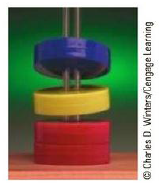Chapter 19, Problem 16CQ

Chapter
Section
Textbook Problem

Figure CQ19.16 shows four permanent magnets, each having a hole through its center. Notice that the blue and yellow magnets are levitated above the red ones. (a) How does this levitation occur? (b) What purpose do the rods serve? (c) What can you say about the poles of the magnets from this observation? (d) If the upper magnet were inverted, what do you suppose would happen?Figure CQ19.16

a)

To determine
How the levitation occurs.

Explanation

Since the blue magnet is levitated, it experiences an upward force due to the presence of the other magnets below it, whose magnitude is equal to the weight of the blue magnet.

The yellow magnet is in between the red magnets and the blue magnets. It experiences a repulsion force due to the red magnets whose magnitude is...

b)

To determine
The purpose of the rods.

c)

To determine
The characters of the poles of the magnets from observation.

d)

To determine
What will happen if the upper magnet is inverted.

Still sussing out bartleby?

Check out a sample textbook solution.

See a sample solution

The Solution to Your Study Problems

Bartleby provides explanations to thousands of textbook problems written by our experts, many with advanced degrees!

Get Started